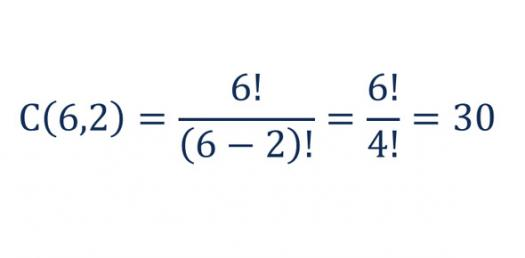# Can You Pass This Factoring Trinomials Test?

10 Questions | Attempts: 1024
ShareSettingsFactoring is a mathematical operation which used to separate down the numbers that multiply together to form another number. Factoring trinomials mean finding two binomials that when multiplied together produce the given trinomial. It uses the same methods for factoring. This quiz has been developed to test your mathematical calculation skills for factoring trinomials. So, let's try out the quiz. All the best! Note: Don't use calculators!

• 1.
3a^2+7a+2
• A.

(a+2)(3a+1)

• B.

(a+1)(3a+2)

• 2.
Factor 6x^2 + 7x + 2
• A.

(3x - 2)(2x -1)

• B.

(2x + 1)(3x + 2)

• C.

(6x + 2)(x +1)

• D.

(2x + 3)(3x -2)

• 3.
Factor 8x^2 + 8x - 6
• A.

2(2x + 1)(2x - 3)

• B.

(2x + 1)(3x + 2)

• C.

2(2x + 3)(2x -1)

• D.

(4x + 1)(2x - 6)

• 4.
Factor 12x^2 - 17x - 5
• A.

(4x + 1)(3x - 5)

• B.

(4x -1)(3x + 5)

• C.

(6x + 1)(2x - 5)

• D.

(2x - 5)(3x + 1)

• 5.
Factor 20x^2 - 46x + 24
• A.

2(2x + 3)(5x - 4)

• B.

(4x - 6)(5x + 4)

• C.

(5x + 4)(4x + 6)

• D.

2(5x - 4)(2x - 3)

• 6.
Factor 6x^2 + 15x + 9
• A.

3(x - 1)(2x - 3)

• B.

3(2x + 3)(x + 1)

• C.

(3x + 1)(2x + 9)

• D.

(2x - 9)(3x - 1)

• 7.
Factor 18t^2 - 24t + 6
• A.

6(3t - 1)(t - 1)

• B.

6(3t + 1)(t + 1)

• C.

3(6t - 1)(t - 1)

• D.

6(t + 1)(3t - 1)

• 8.
Factor 6x^2 + 23x + 7
• A.

(2x - 7)(3x -1)

• B.

(6x + 1)(x + 7)

• C.

(3x + 1)(2x - 7)

• D.

(3x + 1)(2x + 7)

• 9.
Factor 3x^2 + 5x - 2
• A.

(x - 2)(3x + 1)

• B.

(3x - 1)(x - 2)

• C.

(3x - 1)(x + 2)

• D.

(x + 3)(3x - 1)

• 10.
Factor 18x^2 + 3x - 10
• A.

(3x + 2)(6x - 5)

• B.

(6x + 5)(3x + 2)

• C.

6x + 5)(3x - 2)

• D.

(2x + 5)(9x - 2)

## Related TopicsBack to top
×

Wait!
Here's an interesting quiz for you.